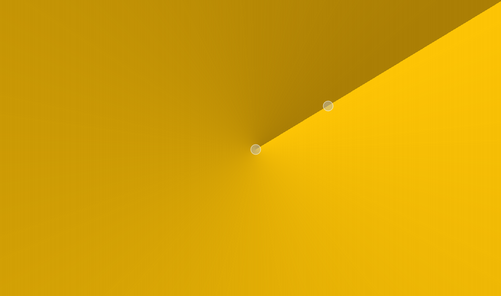The `QConicalGradient` class is used in combination with `QBrush` to specify a conical gradient brush. More

## Detailed Description#

Conical gradients interpolate interpolate colors counter-clockwise around a center point.The colors in a gradient is defined using stop points of the `QGradientStop` type, i.e. a position and a color. Use the `setColorAt()` or the `setStops()` function to define the stop points. It is the gradient’s complete set of stop points that describes how the gradient area should be filled. If no stop points have been specified, a gradient of black at 0 to white at 1 is used.

In addition to the functions inherited from `QGradient` , the `QConicalGradient` class provides the `angle()` and `center()` functions returning the start angle and center of the gradient.

Note that the `setSpread()` function has no effect for conical gradients. The reason is that the conical gradient is closed by definition, i.e. the conical gradient fills the entire circle from 0 - 360 degrees, while the boundary of a radial or a linear gradient can be specified through its radius or final stop points, respectively.

Parameters

Constructs a conical with center at (0, 0) starting the interpolation at angle 0.

Constructs a conical gradient with the given `center`, starting the interpolation at the given `angle`. The `angle` must be specified in degrees between 0 and 360.

Constructs a conical gradient with the given center (`cx`, `cy`), starting the interpolation at the given `angle`. The angle must be specified in degrees between 0 and 360.

Return type

float

Returns the start angle of the conical gradient in logical coordinates.

Return type

`PySide6.QtCore.QPointF`

Returns the center of the conical gradient in logical coordinates.

Parameters

angle – float

Sets `angle` to be the start angle for this conical gradient in logical coordinates.

Sets the center of this conical gradient in logical coordinates to `center`.
Sets the center of this conical gradient in logical coordinates to (`x`, `y`).